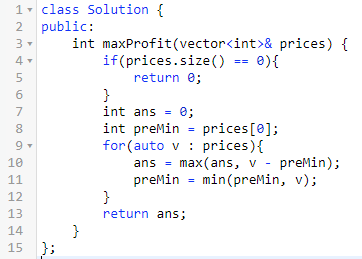# 初级算法实践之动态规划

## 一、背景

1.可以拆分为子问题，并由子问题的答案计算出当前问题的答案。
2.子问题的结果可以保存起来的。

## 二、爬楼梯## 三、买卖股票的最佳时机

`f(n) = max(f(n-1), F(n))`
`F(n) = A[n] - min(n-1)`

`min(n-1)`为前`n-1`天股票的最低价。## 四、最大子序和

`f(n)`可以分两种情况，一种是最后一天属于连续数字，一种是不属于，结果去最优值。
`f(n) = max(f(n-1), F(n))`

`F(n)`也可以分两种情况，一种是自己单独组成连续数字，一种是和前面的连在一起，结果也是取最大值。
`F(n) = max(A[n], A[n] + F(n-1))`

`f(n)`可以拆分为假设每一天为连续数字的最后一个的子问题。
`f(n) = max(F(n), F(n-1), ..., F(1))`## 五、打家劫舍-EOF-

## 关注公众号,接收最新消息

1.## 关注小密圈,学习各种算法

1.